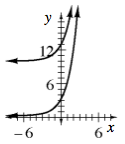### Home > CCA2 > Chapter Ch5 > Lesson 5.2.3 > Problem5-89

5-89.

Graph the two functions below on the same set of axes. Homework Help ✎
$y=3(2^x)\\y=3(2^x)+10$1. How do the two graphs compare?

Look at their y-intercepts.

The second graph is the first, but shifted $10$ units upward.

2. Suppose the first equation is $y=km^x$ and the graph is shifted up $b$ units. What is the new equation?

See part (a).